# Test: Footprints - 1

## 10 Questions MCQ Test Mathematics (Maths) for Class 2 | Test: Footprints - 1

Description
This mock test of Test: Footprints - 1 for Class 2 helps you for every Class 2 entrance exam. This contains 10 Multiple Choice Questions for Class 2 Test: Footprints - 1 (mcq) to study with solutions a complete question bank. The solved questions answers in this Test: Footprints - 1 quiz give you a good mix of easy questions and tough questions. Class 2 students definitely take this Test: Footprints - 1 exercise for a better result in the exam. You can find other Test: Footprints - 1 extra questions, long questions & short questions for Class 2 on EduRev as well by searching above.
QUESTION: 1

### Find the odd one out.

Solution:

This figure does not have any curved line as in the others.

QUESTION: 2

Solution:
QUESTION: 3

### Which shape is 3 in number in the figure given?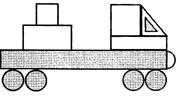Solution:

There are 3 rectangles in the given figure.

QUESTION: 4

The figure given is made up of some squares. How many rectangles are there in it?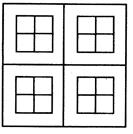Solution:

There are 20 rectangles in the given figure.

QUESTION: 5

Find the missing figure in the pattern.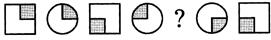Solution:

2nd and 4th squares are the same in the pattern. So, 3rd square must be similar to the 1st square.

QUESTION: 6

How many flat surfaces does the given figure have?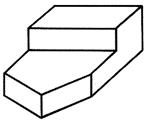Solution:

The given figure has 9 flat surfaces.

QUESTION: 7

The 2 shaded corners of the figure given have been cut out.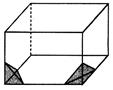How many flat surfaces do each of the 2 corners that have been cut out have?

Solution:

Each of the 2 corners that have been cut out has 4 flat surfaces.

QUESTION: 8

The figure given is made up of some semicircles. How many circles can be made from them?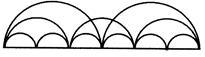Solution:

There are 11 semicircles in the given figure. No. of semicircles needed to make a circle = 2
∴ No. of circles that can be made = 5 (2 × 5 = 10 semicircles. 1 will be left).

QUESTION: 9

How many triangles in the figure are made up of 4 small triangles?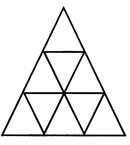Solution:

In the given figure, 3 triangles are made up of 4 small triangles.

QUESTION: 10

Complete the pattern using the correct option given.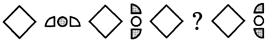Solution:

The quarter circles with a circle in between in 4th figure and 8th figure are mirror images. Similarly, the 6th figure must be the mirror image of the 2nd figure.